# NCERT Solutions for Class 9 Science Chapter 3 - Atoms and Molecules

##### Question 1:

In a reaction, 5.3 g of sodium carbonate reacted with 6 g of ethanoic acid. The products were 2.2 g of carbon dioxide, 0.9 g water and 8.2 g of sodium acetate. Show that these observations are in agreement with the law of conservation of mass.
Sodium carbonate + Ethanoic acid $\to$ Sodium acetate + Carbon dioxide + Water

Mass of sodium carbonate = 5.3 g
Mass of ethanoic acid = 6.0 g
Total mass of the reactants = 5.3 + 6.0
= 11.3 g
Mass of carbon dioxide = 2.2 g
Mass of water = 0.9 g
Mass of sodium ethanoate = 8.2 g
Total mass of the products = 2.2 + 0.9 + 8.2
= 11.3 g
Total mass of the reactants = Total mass of the products Thus, law of conservation of mass is followed.

##### Question 2:

Hydrogen and oxygen combine in the ratio of 1 : 8 by mass to form water. What mass of oxygen gas would be required to react completely with 3 g of hydrogen?

Mass of oxygen gas that reacts with 1 g of hydrogen gas = 8 g
Mass of oxygen gas that reacts with 3 g of oxygen gas = 3 × 8 = 24 g

##### Question 3:

Which postulate of Dalton’s atomic theory is the result of the law of conservation of mass?

Atoms are indivisible particles and can neither be created nor destroyed during any physical or chemical reaction.

##### Question 4:

Which postulate of Dalton’s atomic theory can explain the law of definite proportions?

Atoms of various elements combine in the simple whole number ratio to form compounds.

##### Question 5:

Define the atomic mass unit.

Atomic mass unit is a mass unit and it is equal to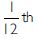of mass of an atom of carbon-12.
1 a.m.u. = 1.66 × 10–27 kg

##### Question 6:

Why is it not possible to see an atom with naked eyes?

This is because atoms are very–very small. The radius of an atom is of the order of 10–10 m.

##### Question 7:

Write down the formulae of
(i) Sodium oxide (ii) Aluminium chloride
(iii) Sodium sulphide (iv) Magnesium hydroxide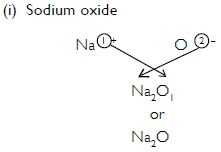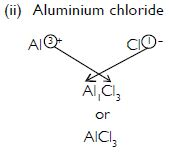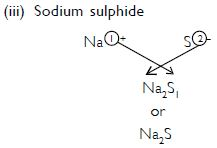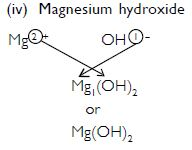##### Question 8:

Write down the names of the compounds represented by the following formulae:
(i) Al2(SO4)3
(ii) CaCl2
(iii) K2SO4
(iv) KNO3
(v) CaCO3

(i) Aluminium sulphate (ii) Calcium chloride
(iii) Potassium sulphate (iv) Potassium nitrate
(v) Calcium carbonate

##### Question 9:

What is meant by the term chemical formula?

Chemical formula: The chemical formula of a substance indicates its constituent elements and number of atoms of each combining element present in one molecule of it.

##### Question 10:

How many atoms are present in a
(i) H2S molecule and

(ii)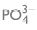(i) An H2S molecule has two atoms of hydrogen and one atom of sulphur.

(ii) A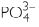ion has one atom of phosphorus and three atoms of oxygen.

##### Question 11:

Calculate the molecular masses of H2 , O2 , Cl2 , CO2 , CH4 , C2 H6 , C2 H4 , NH3 , CH3 OH.

Molecular mass of H2 = 2 × 1 = 2 u
Molecular mass of O2 = 2 × 16 = 32 u
Molecular mass of Cl2 = 2 × 35.5 = 71.0 u
Molecular mass of CO2 = 1 × 12 + 2 × 16 = 12 + 32 = 44 u
Molecular mass of CH4 = 12 + 4 × 1 = 12 + 4 = 16 u
Molecular mass of C2H6 = 2 × 12 + 6 × 1 = 24 + 6 = 30 u
Molecular mass of C2H4 = 2 × 12 + 4 × 1 = 24 + 4 = 28 u
Molecular mass of NH3 = 14 + 3 × 1 = 14 + 3 = 17 u
Molecular mass of CH3OH = 12 + 3 × 1 + 16 + 1 = 12 + 3 + 16 + 1 = 32 u

##### Question 12:

Calculate the formula unit masses of ZnO, Na2O, K2CO3, given atomic masses of Zn = 65 u, Na = 23 u, K = 39 u, C = 12 u and O = 16 u.

Formula unit mass of ZnO = 65 + 16
= 81 u
Formula unit mass of Na2O = 2 × 23 + 16 = 46 + 16 = 62 u
Formula unit mass of K2CO3 = 2 × 39 + 12 + 3 × 16 = 78 + 12 + 48 = 138 u

##### Question 13:

If one mole of carbon atoms weighs 12 gram, what is the mass (in grams) of 1 atom of carbon

1 mole of carbon has mass = 12 g

\ Mass of 1 atom of carbon =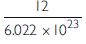= 1.99 × 10–23 g

##### Question 14:

Which has more number of atoms, 100 grams of sodium or 100 grams of iron (Given, atomic mass of Na = 23 u, Fe = 56 u)?

Mass of sodium = 100 g
Atomic mass of sodium = 23 u
$\therefore$ Mass of 1 mole of sodium = 23 g

No. of moles of sodium in 100 g =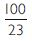$\therefore$ No. of atoms of sodium in 100 g =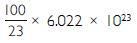=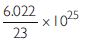= 0.262 × 10–25
= 2.62 × 10–24
Mass of Fe = 100 g
Atomic mass of Fe = 56 u
$\therefore$ Mass of 1 mole of Fe = 56 g

No. of moles of Fe in 100 g =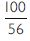=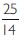No. of atoms of Fe in 100 g =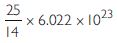=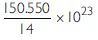= 10.754 × 10–23
= 1.0754 × 10–24
$\therefore$ 100 g of sodium has more number of atoms.

##### Question 15:

A 0.24 g sample of compound of oxygen and boron was found by analysis to contain 0.096 g of boron and 0.144 g of oxygen. Calculate the percentage composition of the compound by weight.

Weight of compound = 0.24 g
Weight of Boron = 0.096 g
Weight of oxygen = 0.144 g

\ % of B =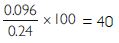% of O =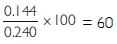##### Question 16:

When 3.0 g of carbon is burnt in 8.00 g oxygen, 11.00 g of carbon dioxide is produced. What mass of carbon dioxide will be formed when 3.00 g of carbon is burnt in 50.00 g of oxygen? Which law of chemical combination will govern your answer?

3.00 g of carbon combines with 8.00 g of oxygen to produce 11.00 g of carbon dioxide.
C + O2 $\to$ CO2
When 3.00 g of carbon is burnt in 50.00 g of oxygen, 11.00 g of carbon dioxide is produced. This is based upon the law of constant compositions.

##### Question 17:

What are polyatomic ions? Give examples.

Polyatomic ion: A group of atoms carrying a charge (+ve or –ve) is called a polyatomic ion.

Examples :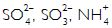##### Question 18:

Write the chemical formulae of the following:
(a) Magnesium chloride (b) Calcium oxide
(c) Copper nitrate (d) Aluminium chloride
(e) Calcium carbonate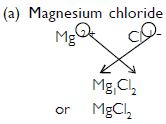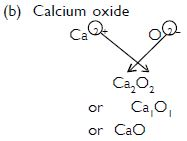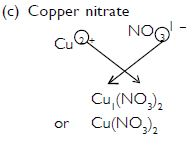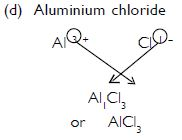##### Question 19:

Give the names of the elements present in the following compounds:
(a) Quick lime (b) Hydrogen bromide
(c) Baking powder (d) Potassium sulphate

(a) Quick lime (CaO): The elements present in it are calcium and oxygen.
(b) Hydrogen bromide (HBr): The elements present in it are hydrogen and bromine.
(c) Baking powder (NaHCO3): The elements present in it are sodium, hydrogen, carbon and oxygen.
(d) Potassium sulphate (K2SO4): The elements present in it are potassium, sulphur and oxygen.

##### Question 20:

Calculate the molar mass of the following substances:
(a) Ethyne, C2H2 (b) Sulphur molecule, S8
(c) Phosphorus molecule, P4 (Atomic mass of phosphorus is 31)
(d) Hydrochloric acid, HCl (e) Nitric acid, HNO3

(a) Molar mass of ethyne, C2H2 = 2 × 12 + 2 × 1 = 24 + 2 = 26 g
(b) Molar mass of sulphur molecule, S8 = 8 × 32 = 256 g
(c) Molar mass of phosphorus molecule, P4 = 4 × 31 = 124 g
(d) Molar mass of hydrochloric acid, HCl = 1 + 35.5 = 36.5 g
(e) Molar mass of nitric acid, HNO3 = 1 + 14 + 3 × 16 = 1 + 14 + 48
= 63 g

##### Question 21:

What is the mass of
(a) 1 mole of nitrogen atom?
(b) 4 moles of aluminium atoms (Atomic mass of aluminium = 27)?
(c) 10 moles of sodium sulphite (Na2SO3)?

(a) Molar mass of ethyne, C2H2 = 2 × 12 + 2 × 1 = 24 + 2 = 26 g
(b) Molar mass of sulphur molecule, S8 = 8 × 32 = 256 g
(c) Molar mass of phosphorus molecule, P4 = 4 × 31 = 124 g
(d) Molar mass of hydrochloric acid, HCl = 1 + 35.5 = 36.5 g
(e) Molar mass of nitric acid, HNO3 = 1 + 14 + 3 × 16 = 1 + 14 + 48
= 63 g

##### Question 22:

What is the mass of
(a) 1 mole of nitrogen atom?
(b) 4 moles of aluminium atoms (Atomic mass of aluminium = 27)?
(c) 10 moles of sodium sulphite (Na2SO3)?

(a) Mass of 1 mole of N atoms = 14 g
(b) Mass of 1 mole of Al atoms = 27 g
$\therefore$ Mass of 4 moles of Al atoms = 4 × 27 = 108 g
(c) Mass of 1 mole of sodium sulphite, Na2SO3
= 2 × 23 + 32 + 3 × 16
= 46 + 32 + 48 = 126 g
$\therefore$ Mass of 10 moles of Na2SO3 = 10 × 126 = 1260 g

##### Question 23:

Convert into moles:
(a) 12 g of oxygen gas
(b) 20 g of water
(c) 22 g of carbon dioxide

We know that, the number moles of a substance, n =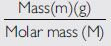(a) No. of moles in 12 g of oxygen gas (O2) =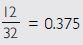(b) No. of moles in 20 g of water (H2O) =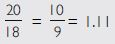(c) No. of moles in 22 g of carbon dioxide (CO2) =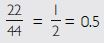##### Question 24:

What is the mass of:
(a) 0.2 mole of oxygen atoms?
(b) 0.5 mole of water molecules?

(a) 0.2 mole of oxygen atoms:
Mass of 1 mole of oxygen atoms = 16 g
Mass of 0.2 mole of oxygen atoms = 0.2 × 16 = 3.2 g
(b) 0.5 mole of water molecules:
Mass of 1 mole of water molecule (H2O) = 18 g
$\therefore$ Mass of 0.5 mole of water molecules = 0.5 × 18 = 9 g

##### Question 25:

Calculate the number of molecules of sulphur (S8) present in 16 g of solid sulphur.

Mass of solid sulphur (S8) = 16 g
Mass of 1 mole of sulphur (S8) = 8 × 32 = 256 g

No. of atoms in 16 g of solid sulphur =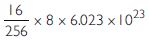= 3.0115 × 1023

##### Question 26:

Calculate the number of aluminium ions present in 0.051 g of aluminium oxide.